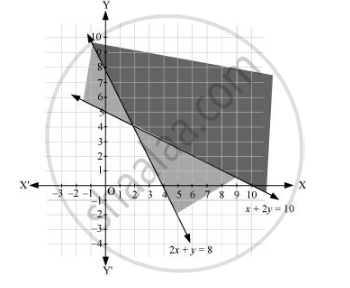CBSE (Arts) Class 11CBSE
Share

# Solve the Following System of Inequalities Graphically: 2x + Y≥ 8, X + 2y ≥ 10 - CBSE (Arts) Class 11 - Mathematics

ConceptSolution of System of Linear Inequalities in Two Variables

#### Question

Solve the following system of inequalities graphically: 2x + y≥ 8, x + 2y ≥ 10

#### Solution

2x + y= 8 … (1)

x + 2= 10 … (2)

The graph of the lines, 2x + y= 8 and x + 2= 10, are drawn in the figure below.

Inequality (1) represents the region above the line, 2x + = 8, and inequality (2) represents the region above the line, x + 2= 10.

Hence, the solution of the given system of linear inequalities is represented by the common shaded region including the points on the respective lines as follows.Is there an error in this question or solution?

#### Video TutorialsVIEW ALL 

Solution Solve the Following System of Inequalities Graphically: 2x + Y≥ 8, X + 2y ≥ 10 Concept: Solution of System of Linear Inequalities in Two Variables.
S